# Secant-cubed function

(Redirected from Sec^3)
This article is about a particular function from a subset of the real numbers to the real numbers. Information about the function, including its domain, range, and key data relating to graphing, differentiation, and integration, is presented in the article.
View a complete list of particular functions on this wiki
For functions involving angles (trigonometric functions, inverse trigonometric functions, etc.) we follow the convention that all angles are measured in radians. Thus, for instance, the angle of$90\,^\circ$ is measured as$\pi/2$.

## Definition

The function, denoted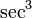$\sec^3$, is defined as the composite of the cube function and the secant function (which in turn is the composite of the reciprocal function and the cosine function). Explicitly, it is given as:$x \mapsto (\sec x)^3 = \frac{1}{(\cos x)^3}$

## Differentiation

### First derivative

We can differentiate this function as a composite function, using the chain rule for differentiation. We get: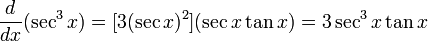$\! \frac{d}{dx}(\sec^3x) = [3(\sec x)^2](\sec x \tan x) = 3 \sec^3x \tan x$

Alternatively, we could consider it as$1/(\cos x)^3$, and hence as a composite of$t \mapsto 1/t^3$ and$\cos$. With that interpretation, the chain rule for differentiation yields: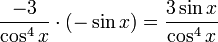$\! \frac{-3}{\cos^4x} \cdot (-\sin x) = \frac{3\sin x}{\cos^4 x}$

The two answers can be verified to be equivalent.

## Integration

### First antiderivative

We use the recursive version of integration by parts.

We rewrite$\sec^3x = \sec x \cdot \sec^2x$ and perform integration by parts, taking$\sec^2$ as the part to integrate. We use that an antiderivative of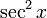$\sec^2 x$ is$\tan x$ whereas the derivative of$\sec x$ is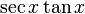$\sec x \tan x$: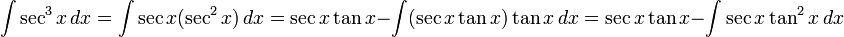$\int \sec^3 x \, dx = \int \sec x (\sec^2 x) \,dx = \sec x \tan x - \int (\sec x \tan x) \tan x \, dx = \sec x \tan x - \int \sec x \tan^2x \, dx$

We now use the fact that$\tan^2 x + 1 = \sec^2 x$, or more explicitly,$\tan^2 x = \sec^2 x - 1$, to rewrite this as:$\! \int \sec^3 x \, dx = \sec x \tan x - \int \sec x (\sec^2 x - 1) \, dx = \sec x \tan x x - \int \sec^3 x \, dx + \int \sec x \, d$

We now use the integration of the secant function to simplify this as: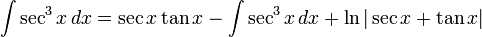$\! \int \sec^3 x \, dx = \sec x \tan x - \int \sec^3 x \, dx + \ln|\sec x + \tan x|$

We can choose an antiderivative$I$ of$\sec^3$ so that the above equality (between the left-most and right-most expression) holds without any additive constant adjustment, and we get: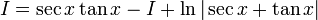$\! I = \sec x \tan x - I + \ln|\sec x + \tan x|$

We rearrange and obtain:$2I = \sec x \tan x + \ln|\sec x + \tan x|$

Dividing by 2, we get:$\! I = \frac{\sec x \tan x + \ln|\sec x + \tan x|}{2}$

The general antiderivative expression is thus: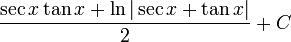$\frac{\sec x \tan x + \ln|\sec x + \tan x|}{2} + C$